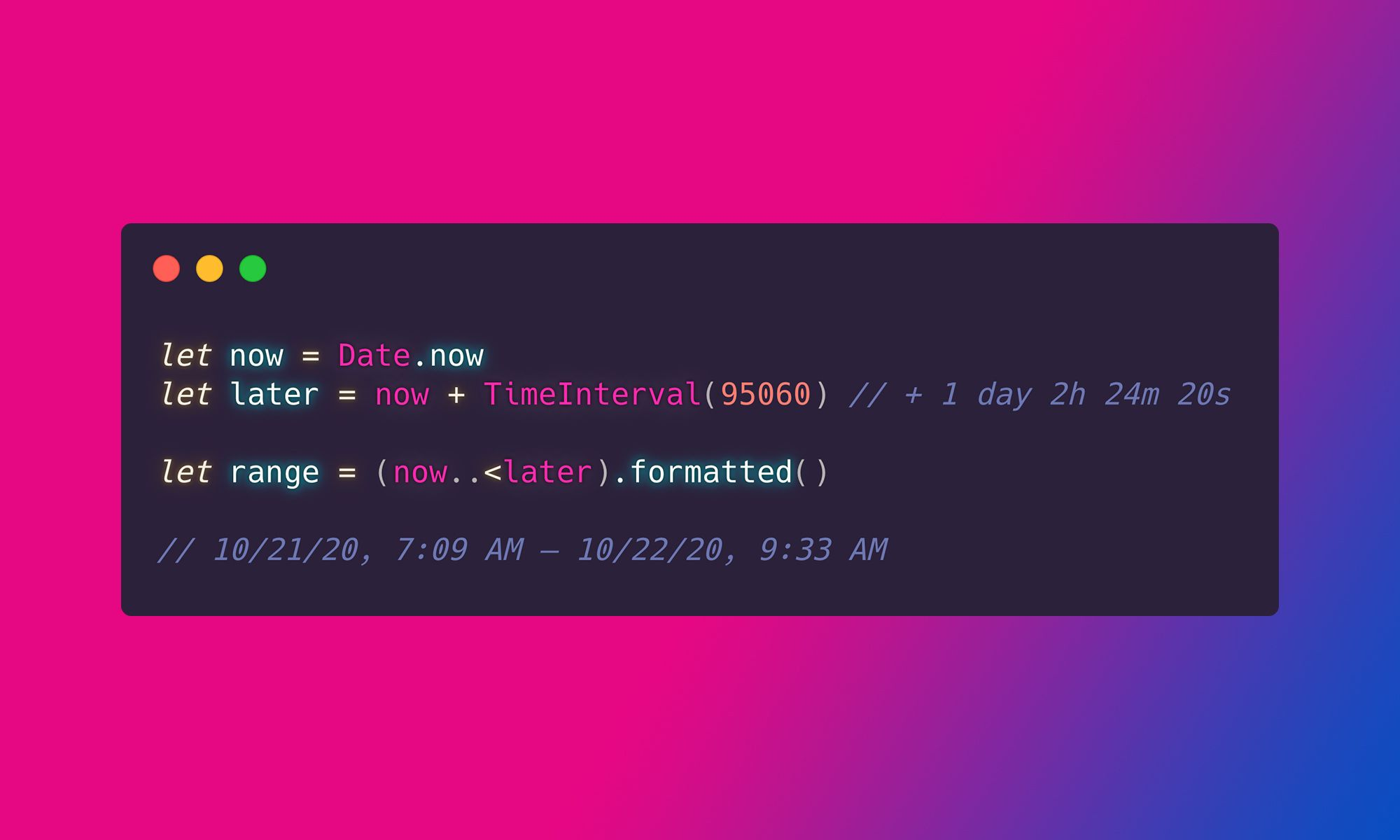# How to format a Time Interval in iOS 15 and macOS 12

During WWDC 2021, Apple introduced the new APIs that make Time Interval formatting a lot easier. Without further ado, let's see how to display the time range using the `formatted()` method:

``````let now = Date.now
let later = now + TimeInterval(95060) // + 1 day 2h 24m 20s

let range = (now..<later).formatted()

// 10/21/20, 7:09 AM – 10/22/20, 9:33 AM``````

By default, it will return the time interval with both date and time components. Like with date formatting,  we can skip the component we don't need:

``````let now = Date.now
let later = now + TimeInterval(95060) // + 1 day 2h 24m 20s

let noDate = (now..<later).formatted(date: .numeric, time: .omitted)

// 10/21/20 – 10/22/20``````
Although, at the moment of writing this article, it looks like we cannot skip the `.date` part using this method. Not sure whether this is intentional behavior or a bug.

Of course, we can customize the returned components:

``````let now = Date.now
let later = now + TimeInterval(95060) // + 1 day 2h 24m 20s

let customized = (now..<later).formatted(date: .abbreviated, time: .complete)

Oct 21, 2020, 7:26:00 AM GMT+2 – Oct 22, 2020, 9:50:20 AM GMT+2``````

If we want to display the duration of the Time Interval we  can use the `.timeDuration` style:

``````let now = Date.now
let later = now + TimeInterval(95060) // + 1 day 2h 24m 20s

let timeDuration = (now..<later).formatted(.timeDuration)
// 26:24:20``````

The `.timeDuration` displays the Time Interval as hours, minutes, and seconds. If we also need days, months, etc., we can use the `.components`  style:

``````let now = Date.now
let later = now + TimeInterval(95060) // + 1 day 2h 24m 20s

let components = (now..<later).formatted(.components(style: .wide))
// 1 day, 2 hours, 24 minutes, 20 seconds``````

To display the relative difference between dates, we can use the `.relative` style:

``````let now = Date.now
let later = now + TimeInterval(95060) // + 1 day 2h 24m 20s

let relative = later.formatted(.relative(presentation: .numeric, unitsStyle: .wide))
// in 1 day``````

The code above will  show the `.numeric` difference, but for a longer Time Interval we might want a `.named` version:

``````let now = Date.now
let later = now + TimeInterval(95060) // + 1 day 2h 24m 20s

let relative = later.formatted(.relative(presentation: .named, unitsStyle: .wide))
// tomorrow``````

That's it. I hope this short article was useful. If so, check out my other article where I explain how to format a Date: